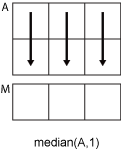# median

## 语法

``M = median(A)``
``M = median(A,"all")``
``M = median(A,dim)``
``M = median(A,vecdim)``
``M = median(___,missingflag)``

## 说明

````M = median(A)` 返回 `A` 的中位数值。 如果 `A` 为向量，则 `median(A)` 返回 `A` 的中位数值。如果 `A` 为非空矩阵，则 `median(A)` 将 `A` 的各列视为向量，并返回中位数值的行向量。如果 `A` 为 0×0 空矩阵，`median(A)` 返回 `NaN`。如果 `A` 为多维数组，则 `median(A)` 将沿大小大于 `1` 的第一个数组维度的值视为向量。此维度中 `M` 的大小变为 `1`，而所有其他维度的大小仍与在 `A` 中相同。如果 `A` 是表或时间表，则 `median(A)` 返回包含每个变量的中位数的单行表。 （自 R2023a 起） `median` 固有地以与 `A` 相同的类返回值，使得 `class(M) = class(A)`。```

``M = median(A,"all")` 返回 `A` 的所有元素的中位数。`

``M = median(A,dim)` 返回维度 `dim` 上元素的中位数。例如，如果 `A` 为矩阵，则 `median(A,2)` 返回包含每一行的中位数值的列向量。`

``M = median(A,vecdim)` 返回向量 `vecdim` 所指定的维度上的中位数。例如，如果 `A` 是矩阵，则 `median(A,[1 2])` 返回 `A` 中所有元素的中位数，因为矩阵的每个元素包含在由维度 1 和 2 定义的数组切片中。`

``M = median(___,missingflag)` 在上述任一语法的基础上指定包含还是省略 `A` 中的缺失值。例如，`median(A,"omitmissing")` 在计算中位数时会忽略所有缺失值。默认情况下，`median` 包括缺失值。`

## 示例

`A = [0 1 1; 2 3 2; 1 3 2; 4 2 2]`
```A = 4×3 0 1 1 2 3 2 1 3 2 4 2 2 ```

`M = median(A)`
```M = 1×3 1.5000 2.5000 2.0000 ```

`A = [0 1 1; 2 3 2]`
```A = 2×3 0 1 1 2 3 2 ```

`M = median(A,2)`
```M = 2×1 1 2 ```

```rng('default') A = randi(10,[1,3,4])```
```A = A(:,:,1) = 9 10 2 A(:,:,2) = 10 7 1 A(:,:,3) = 3 6 10 A(:,:,4) = 10 2 10 ```

`M = median(A)`
```M = M(:,:,1) = 9 M(:,:,2) = 7 M(:,:,3) = 6 M(:,:,4) = 10 ```

```M = median(A,1); isequal(A,M)```
```ans = logical 1 ```

```A(:,:,1) = [2 4; -2 1]; A(:,:,2) = [6 2; -5 3]; A(:,:,3) = [4 4; 7 -3]; M1 = median(A,[1 2])```
```M1 = M1(:,:,1) = 1.5000 M1(:,:,2) = 2.5000 M1(:,:,3) = 4 ```

`M2 = median(A,[1 2 3])`
```M2 = 2.5000 ```
`Mall = median(A,"all")`
```Mall = 2.5000 ```

`A = int8(1:4)`
```A = 1x4 int8 row vector 1 2 3 4 ```

`M = median(A)`
```M = int8 3 ```
`class(M)`
```ans = 'int8' ```

`M` 为在排列顺序上位于中间的两个数值的均值（以一个 8 位整数返回）。

`A = [1.77 -0.005 NaN -2.95; NaN 0.34 NaN 0.19]`
```A = 2×4 1.7700 -0.0050 NaN -2.9500 NaN 0.3400 NaN 0.1900 ```

`M = median(A,"omitmissing")`
```M = 1×4 1.7700 0.1675 NaN -1.3800 ```

## 输入参数

• `median(A,1)` 计算 `A` 的每列中元素的中位数，并返回一个 `1`×`n` 行向量。• `median(A,2)` 计算 `A` 的每行中元素的中位数，并返回一个 `m`×`1` 列向量。`dim` 大于 `ndims(A)` 时，`median` 返回 `A``"includemissing"`所有支持的数据类型

`"includenan"``double`, `single`, `duration`
`"includenat"``datetime`
`"includeundefined"``categorical`
`"omitmissing"`所有支持的数据类型忽略 `A` 中的缺失值并基于较少的点计算中位数。如果运算维度中的所有元素都缺失，则 `M` 中的对应元素也会缺失。
`"omitnan"``double`, `single`, `duration`
`"omitnat"``datetime`
`"omitundefined"``categorical`